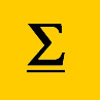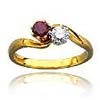# Search by Topic

#### Resources tagged with Binomial Theorem similar to Tens:

Filter by: Content type:
Age range:
Challenge level:

### There are 11 results

Broad Topics > Algebraic expressions, equations and formulae > Binomial Theorem### Tens

##### Age 16 to 18 Challenge Level:

When is $7^n + 3^n$ a multiple of 10? Can you prove the result by two different methods?### Elevens

##### Age 16 to 18 Challenge Level:

Add powers of 3 and powers of 7 and get multiples of 11.### The Harmonic Triangle and Pascal's Triangle

##### Age 16 to 18

The harmonic triangle is built from fractions with unit numerators using a rule very similar to Pascal's triangle.### Telescoping Series

##### Age 16 to 18 Challenge Level:

Find $S_r = 1^r + 2^r + 3^r + ... + n^r$ where r is any fixed positive integer in terms of $S_1, S_2, ... S_{r-1}$.### Growing

##### Age 16 to 18 Challenge Level:

Which is larger: (a) 1.000001^{1000000} or 2? (b) 100^{300} or 300! (i.e.factorial 300)### Summit

##### Age 16 to 18 Challenge Level:

Prove that the sum from t=0 to m of (-1)^t/t!(m-t)! is zero.### Remainder Hunt

##### Age 16 to 18 Challenge Level:

What are the possible remainders when the 100-th power of an integer is divided by 125?### The Kth Sum of N Numbers

##### Age 16 to 18

Yatir from Israel describes his method for summing a series of triangle numbers.### Bina-ring

##### Age 16 to 18 Challenge Level:

Investigate powers of numbers of the form (1 + sqrt 2).### Discrete Trends

##### Age 16 to 18 Challenge Level:

Find the maximum value of n to the power 1/n and prove that it is a maximum.### Binomial

##### Age 16 to 18 Challenge Level:

By considering powers of (1+x), show that the sum of the squares of the binomial coefficients from 0 to n is 2nCn